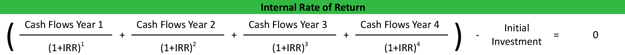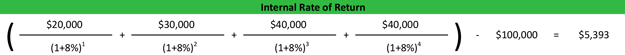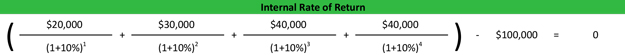# Internal Rate of Return (IRR)

Internal rate of return (IRR) is the minimum discount rate that management uses to identify what capital investments or future projects will yield an acceptable return and be worth pursuing. The IRR for a specific project is the rate that equates the net present value of future cash flows from the project to zero. In other words, if we computed the present value of future cash flows from a potential project using the internal rate as the discount rate and subtracted out the original investment, our net present value of the project would be zero.

## Definition – What is the Internal Rate of Return Ratio?

This sounds a little confusing at first, but it’s pretty simple. Think of it in terms of capital investing like the company’s management would. They want to calculate what percentage return is required to break even on an investment adjusted for the time value of money. You can think of the internal rate of return as the interest percentage that company has to achieve in order to break even on its investment in new capital. Since management wants to do better than break even, they consider this the minimum acceptable return on an investment.

## IRR Formula

The IRR formula is calculated by equating the sum of the present value of future cash flow less the initial investment to zero. Since we are dealing with an unknown variable, this is a bit of an algebraic equation. Here’s what it looks like:As you can see, the only variable in the internal rate of return equation that management won’t know is the IRR. They will know how much capital is required to start the project and they will have a reasonable estimate of the future income of the investment. This means we will have solve for the discount rate that will make the NPV equal to zero.

## Example of Calculating IRR

It might be easier to look at an example than to keep explaining it. Let’s look at Tom’s Machine Shop. Tom is considering purchasing a new machine, but he is unsure if it’s the best use of company funds at this point in time. With the new \$100,000 machine, Tom will be able to take on a new order that will pay \$20,000, \$30,000, \$40,000, and \$40,000 in revenue.

Let’s calculate Tom’s minimum rate. Since it’s difficult to isolate the discount rate unless you use an excel IRR calculator. You can start with an approximate rate and adjust from there. Let’s start with 8 percent.As you can see, our ending NPV is not equal to zero. Since it’s a positive number, we need to increase the estimated internal rate. Let’s increase it to 10 percent and recalculate.As you can see, Tom’s internal return rate on this project is 10 percent. He can compare this to other investing opportunities to see if it makes sense to spend \$100,000 on this piece of equipment or investment the money in another venture.

## Internal Rate of Return Analysis

Remember, IRR is the rate at which the net present value of the costs of an investment equals the net present value of the expected future revenues of the investment. Management can use this return rate to compare other investments and decide what capital projects should be funded and what ones should be scrapped.

Going back to our machine shop example, assume Tom could purchase three different pieces of machinery. Each would be used for a slightly different job that brought in slightly different amounts of cash flow. Tom can calculate the internal rate of return on each machine and compare them all. The one with the highest IRR would be the best investment.

Since this is an investment calculation, the concept can also be applied to any other investment. For instance, Tom can compare the return rates of investing the company’s money in the stock market or new equipment. Now obviously the expected future cash flows aren’t always equal to the actual cash received in the future, but this represents a starting point for management to base their purchase and investment decisions on.